# Shift Register (Left)

The Shift Register (Left) models a combination of a clocked data type register and a shift register with left shifts only. The clock edge for the register can be set with the Trigger Condition parameter to be a rising edge (0_TO_1) or a falling edge (1_TO_0). The set/reset events can be configured to be asynchronous or synchronous to the clock with the Set/Reset Type parameter. The active logic level of the inputs can be configured with the Set/Reset Level and Load/Shift Level parameters.

For the left shift register with both asynchronous and synchronous set and reset inputs, see Shift Register (Left) with both Sync and Async Set/Resetkeyword.

Related topics:

In this topic:

Model Name: Shift Register (Left)
Simulator: This device is compatible with the SIMPLIS simulator.
Parts Selector Menu Location: Digital Functions > Registers
Symbol Library: None - the symbol is automatically generated when placed or edited.
Model Library: None - the model is automatically generated when the simulation is run.
Subcircuit Names:
• SIMPLIS_DIGI1_D_SHIFT_REG_LEFT_SOAS_N : Without Ground Reference
• SIMPLIS_DIGI1_D_SHIFT_REG_LEFT_SOAS_Y : With Ground Reference
Symbol: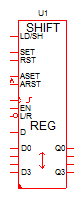4-bit, rising edge triggered, synchronous set/reset, without ground reference.
Multiple Selections: Only one device at a time can be edited.

## Editing the Shift Register (Left)

To configure the Shift Register (Left), follow these steps:

1. Double click the symbol on the schematic to open the editing dialog to the Parameters tab.
2. Make the appropriate changes to the fields described in the table below the image.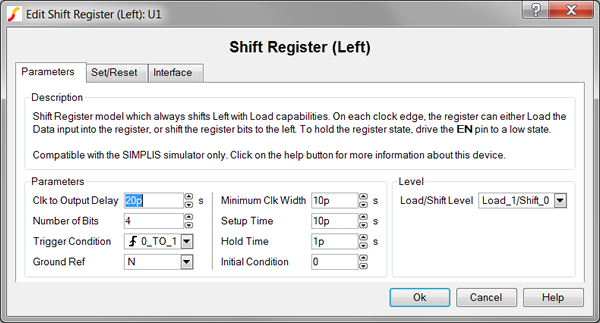Label Parameter Description Clock to Output Delay Delay from the triggering clock event until the Register outputs change Number of Bits Number of input bits to the Register Trigger Condition Determines the triggering condition of the Register clock pin: 0_TO_1 for rising edge triggered 1_TO_0 for falling edge triggered Ground Ref Determines whether or not a device has a ground reference pin. Any digital component that has an input or output pin connected to an analog circuit node must have its Ground Ref pin connected to an analog node. This is usually the ground on the schematic. Minimum Clk Width Minimum valid clock width. Clock widths less than this parameter will not trigger the Register. Setup Time Minimum time before the triggering clock event that the input signals must remain steady so that a valid change in each input state is recognized. Hold Time Minimum time after the triggering clock event that the input signals must remain steady so that a valid change in each input state is recognized. Initial Condition Initial condition of the Register output at time=0 Load/Shift Level Determines the logic level of the shift register load/shift ( LD/SH ) pin: Load_0/Shift_1 means load on low and shift on high. Load_1/Shift_0 means load on high and shift on low.

To define the parameters for the Set/Reset, follow these steps:

1. From the Edit Shift Register (Left) dialog box, click on the Set/Reset tab.
2. Make the appropriate changes to the fields described in the table below the image.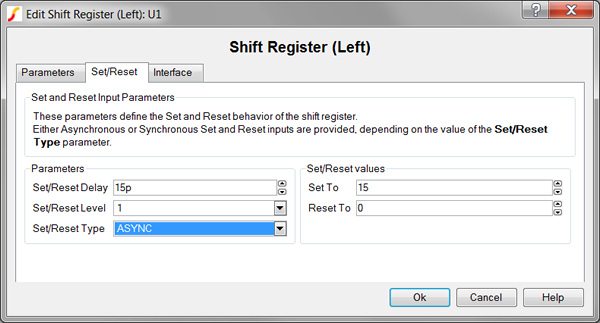Label Parameter Description
Set/Reset Delay Delay from when the SET or RST pin goes active until the Q output is actually set or reset.
Set/Reset Level Determines the Set/Reset level of a device:
• 1 means active high
• 0 means active low
Set/Reset Type Determines whether or not output events are synchronized with a clock event:
 Set/Reset Type Description SYNC Set/reset events are synchronized to the clock edge defined by the Trigger Condition parameter. ASYNC Set/reset events are asynchronous to the clock edge.
.
Set To Determines the value of the counter output when the SET pin goes active. To set to the maximum count value, assign a value of -1.
Reset To Determines the value of the counter output when the RST pin goes active. To reset to 0, assign a assign value of -1 or 0.

To define the parameters for the interface between this digital component and each analog component connected directly to an input or output pin, follow these steps:

1. From the Edit Shift Register (Left) dialog box, click on the Interface tab.
2. Make the appropriate changes to the fields described in the table below the image.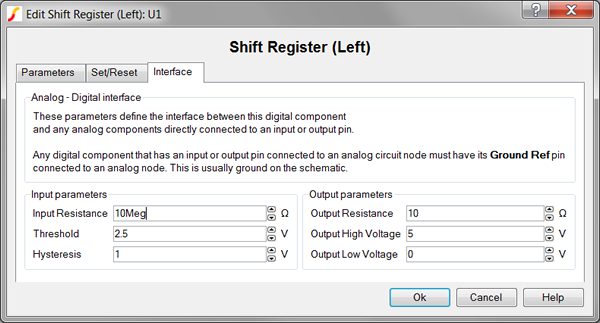Label Parameter Description
Input Resistance Input resistance of each Register input pin
Hysteresis, Threshold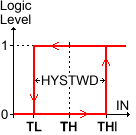Hysteresis and Threshold of the inputs. The hysteretic-window width, HYSTWD is centered around Threshold (TH) voltage. To determine the actual threshold ( TL , THI ), substitute Threshold (TH) and Hysteresis (HYSTWD) in each of the following formulas:
 Input Logic Level Actual Threshold 1 Threshold + 0.5 * Hysteresis 0 Threshold - 0.5 * Hysteresis
Output Resistance Output resistance of each Register output pin
Output High Voltage Output high voltage for each Register output pin
Output Low Voltage Output high voltage for each Register output pin

## Truth Tables

The truth tables below show the logic behavior with asynchronous and synchronous set/reset

### Asynchronous Set/Reset

The following truth table assumes these parameter values:

• Set/Reset Type=ASYNC
• Trigger Condition=0_TO_1 which represents a rising edge, clocked, left shift register
• Set/Reset level=1

When the EN input is high and the Load/Shift Level=Load_1/Shift_0, the register will load the D input when the LD/SH pin is high and shift the Q output when the LD/SH pin is low.

 Inputs Output Action LD/SH ASET ARST EN D CLK Q 0 or 1 0 0 0 0 or 1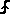Last Q Retain state 1 0 0 1 0 or 1Data input Load data 0 0 0 1 0Q = Last Q shifted left, LSB=0 Shift left 0 0 0 1 1Q = Last Q shifted left, LSB=1 Shift left 0 or 1 1 0 0 or 1 0 or 1 0 or 1 Asynchronous Set To value Asynchronous set 0 or 1 0 1 0 or 1 0 or 1 0 or 1 Asynchronous Reset To value Asynchronous reset 0 or 1 1 1 0 or 1 0 or 1 0 or 1 Last Q Illegal concurrent ASET and ARST

### Synchronous Set/Reset

The following truth table assumes these parameter values:

• Set/Reset Type=SYNC
• Trigger Condition=0_TO_1 which represents a rising edge, clocked, left shift register
• Set/Reset level=1

When the EN input is high and the Load/Shift Level=Load_1/Shift_0, the register will load the D input when the LD/SH pin is high and shift the Q output when the LD/SH pin is low.

 Inputs Output Action LD/SH ASET ARST EN D CLK Q 0 or 1 0 0 0 0 or 1Last Q Retain state 1 0 0 1 0 or 1Data input Load data 0 0 0 1 0Q = Last Q shifted left, LSB=0 Shift left 0 0 0 1 1Q = Last Q shifted left, LSB=1 Shift left 0 or 1 1 0 0 or 1 0 or 1Synchronous Set To value Synchronous set 0 or 1 0 1 0 or 1 0 or 1Synchronous Reset To value Synchronous reset 0 or 1 1 1 0 or 1 0 or 1Last Q Illegal concurrent SET and RST

## Examples

The test circuit used to generate the waveform examples in the next section can be downloaded here: simplis_052_shiftregisterleftsoas_example.zip.

To simulate this design, follow these steps:

1. Unzip the archive to a location on your computer.
2. To open the schematic, double click the .sxsch file or drag that file into the SIMetrix/SIMPLIS Command Shell.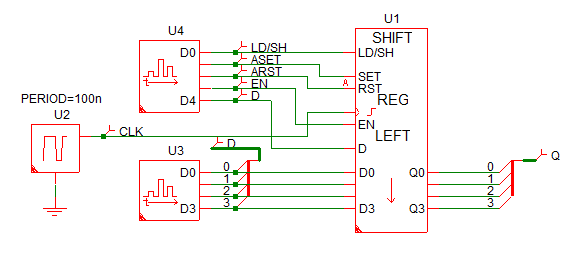## Waveforms

This example of the left shift register uses two Digital Signal Source to generate the input pulses to the left shift register. The input source waveforms are defined in a text file with each state transition defined on a single line. These two sources excite every state transition in the Truth Table section.

For clarity, the simulation waveforms taken from the circuit example have been divided into three sections.

• Load and Shift Operations when D=0: 0-1us
• Load and Shift Operations when D=1: 1-2us
• Asynchronous and Synchronous Set/Reset: 2-3us

### Load and Shift Operations when D=0: 0-1us

The image below shows the load and shift behavior when the D input is 0. During a Left shift operation, the D input is shifted into the LSB; since D=0, the new Q output is twice the old Q. The initial condition of the left shift register is set to 15 decimal in the example.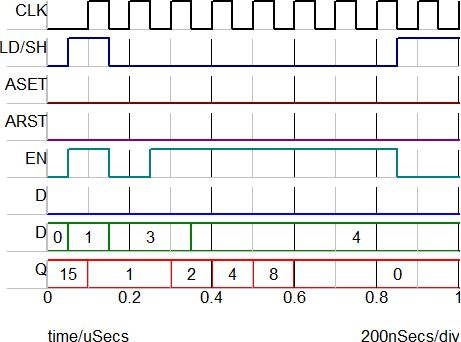Time Event Q Output 100n Load 1 200n Retain state 1 300n Shift left 2 400n Shift left 4 500n Shift left 8 600n Shift left 0 700n Shift left 0 800n Shift left 0

### Load and Shift Operations when D=1: 1-2us

The image below shows the load and shift behavior when the D input is 1. During a Left shift operation, the D input is shifted into the LSB, effectively adding one to the result.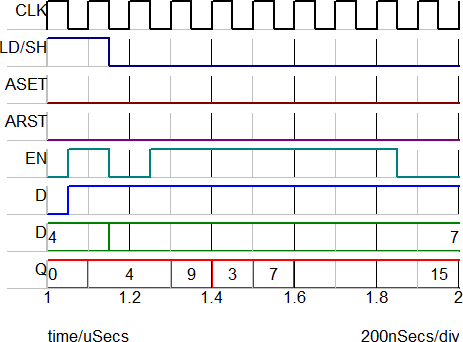Time Event Q Output 1.1u Load 4 1.2u Retain state 4 1.3u Shift left 9 1.4u Shift left 3 1.5u Shift left 7 1.6u Shift left 15 1.7u Shift left 15 1.8u Shift left 15

### Asynchronous Set/Reset: 2-3us

The image below shows the asynchronous set/reset behavior of the register.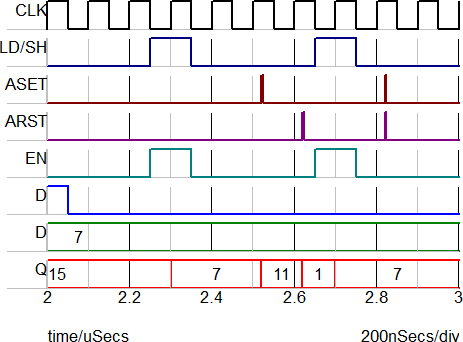Asynchronous and Synchronous Set/Reset

 Time Event Q Output 2.3u Load 7 2.52u Asynchronous set 11 2.62u Asynchronous reset 1 2.7u Load 7 2.82u Illegal concurrent ASET and ARST 7

## Subcircuit Parameters

Because the Shift Register (Left) model is generated by a template script when the simulation is executed, a hand-coded model cannot be inserted into a netlist. The template script for this device is simplis_make_register_model.sxscr, which licensed users can download as part of a zip archive of all built-in scripts.

The following parameter table defines the parameters used in this model.

Parameter Name Label Data Type Range Units Parameter Description
CLK_TO_OUT_DELAY Clock to Output Delay Number 1f to 1024 s Delay from the triggering clock event until the Register outputs change
GNDREF Ground Ref String
• 'Y'
• 'N'
none Determines whether or not a device has a ground reference pin. Any digital component that has an input or output pin connected to an analog circuit node must have its Ground Ref pin connected to an analog node. This is usually the ground on the schematic.
HOLD_TIME Hold Time Number 1f to 1024 s Minimum time after the triggering clock event that the input signals must remain steady so that a valid change in each input state is recognized.
HYSTWD,
TH
Hysteresis,
Threshold
Number min: 1f VHysteresis and Threshold of the inputs. The hysteretic-window width, HYSTWD is centered around Threshold (TH) voltage. To determine the actual threshold ( TL , THI ), substitute Threshold (TH) and Hysteresis (HYSTWD) in each of the following formulas:
 Input Logic Level Actual Threshold 1 Threshold + 0.5 * Hysteresis 0 Threshold - 0.5 * Hysteresis
IC Initial Condition Number
• 0
• 1
none Initial condition of the Register output at time=0
none Determines the logic level of the shift register load/shift ( LD/SH ) pin:
MIN_CLK Minimum Clk Width Number 1f to 1024 s Minimum valid clock width. Clock widths less than this parameter will not trigger the Register.
NUMBITS Number of Bits Integer   none Number of input bits to the Register
RESET_TO Reset To Number   none Determines the value of the counter output when the RST pin goes active. To reset to 0, assign a assign value of -1 or 0.
RIN Input Resistance Number min: 100 Input resistance of each Register input pin
ROUT Output Resistance Number min: 1m Output resistance of each Register output pin
SETUP_TIME Setup Time Number 1f to 1024 s Minimum time before the triggering clock event that the input signals must remain steady so that a valid change in each input state is recognized.
SET_RESET_DELAY Set/Reset Delay Number 1f to 1024 s Delay from when the SET or RST pin goes active until the Q output is actually set or reset.
SET_RESET_LEVEL Set/Reset Level Number
• 0
• 1
none Determines the Set/Reset level of a device:
• 1 means active high
• 0 means active low
SET_RESET_TYPE Set/Reset Type String
• 'SYNC'
• 'ASYNC'
none Determines whether or not output events are synchronized with a clock event:
 Set/Reset Type Description SYNC Set/reset events are synchronized to the clock edge defined by the Trigger Condition parameter. ASYNC Set/reset events are asynchronous to the clock edge.
.
SET_TO Set To Number   none Determines the value of the counter output when the SET pin goes active. To set to the maximum count value, assign a value of -1.
TRIG_COND Trigger Condition String
• '0_TO_1'
• '1_TO_0'
none Determines the triggering condition of the Register clock pin:
• 0_TO_1 for rising edge triggered
• 1_TO_0 for falling edge triggered
VOH Output High Voltage Number any V Output high voltage for each Register output pin
VOL Output Low Voltage Number any V Output high voltage for each Register output pin